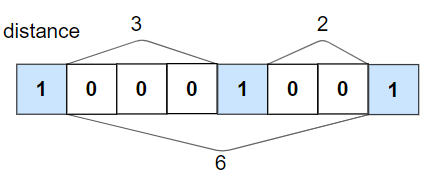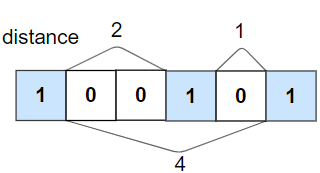## 题目

### A. 旅行终点站

• 1 <= paths.length <= 100
• paths[i].length == 2
• 1 <= cityAi.length, cityBi.length <= 10
• cityAi != cityBi
• 所有字符串均由大小写英文字母和空格字符组成。

### B. 是否所有 1 都至少相隔 k 个元素• 1 <= nums.length <= 10^5
• 0 <= k <= nums.length
• nums[i] 的值为 01

### C. 绝对差不超过限制的最长连续子数组

• 1 <= nums.length <= 10^5
• 1 <= nums[i] <= 10^9
• 0 <= limit <= 10^9

### D. 有序矩阵中的第 k 个最小数组和

• m == mat.length
• n == mat.length[i]
• 1 <= m, n <= 40
• 1 <= k <= min(200, n ^ m)
• 1 <= mat[i][j] <= 5000
• mat[i] 是一个非递减数组